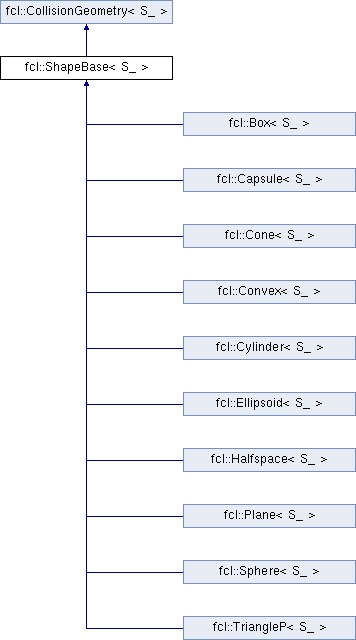FCL  0.6.0 Flexible Collision Library
fcl::ShapeBase< S_ > Class Template Reference

Base class for all basic geometric shapes. More...

`#include <shape_base.h>`

Inheritance diagram for fcl::ShapeBase< S_ >:using S = S_

## Public Member Functions

OBJECT_TYPE getObjectType () const
Get object type: a geometric shape.Public Member Functions inherited from fcl::CollisionGeometry< S_ >
virtual NODE_TYPE getNodeType () const
get the node type

virtual void computeLocalAABB ()=0
compute the AABB for object in local coordinate

void * getUserData () const
get user data in geometry

void setUserData (void *data)
set user data in geometry

bool isOccupied () const
whether the object is completely occupied

bool isFree () const
whether the object is completely free

bool isUncertain () const
whether the object has some uncertainty

virtual Vector3< S_ > computeCOM () const
compute center of mass

virtual Matrix3< S_ > computeMomentofInertia () const
compute the inertia matrix, related to the origin

virtual S_ computeVolume () const
compute the volume

virtual Matrix3< S_ > computeMomentofInertiaRelatedToCOM () const
compute the inertia matrix, related to the comPublic Attributes inherited from fcl::CollisionGeometry< S_ >
Vector3< S_ > aabb_center
AABB center in local coordinate.

AABB< S_ > aabb_local
AABB in local coordinate, used for tight AABB when only translation transform.

void * user_data
pointer to user defined data specific to this object

S_ cost_density
collision cost for unit volume

S_ threshold_occupied
threshold for occupied ( >= is occupied)

S_ threshold_free